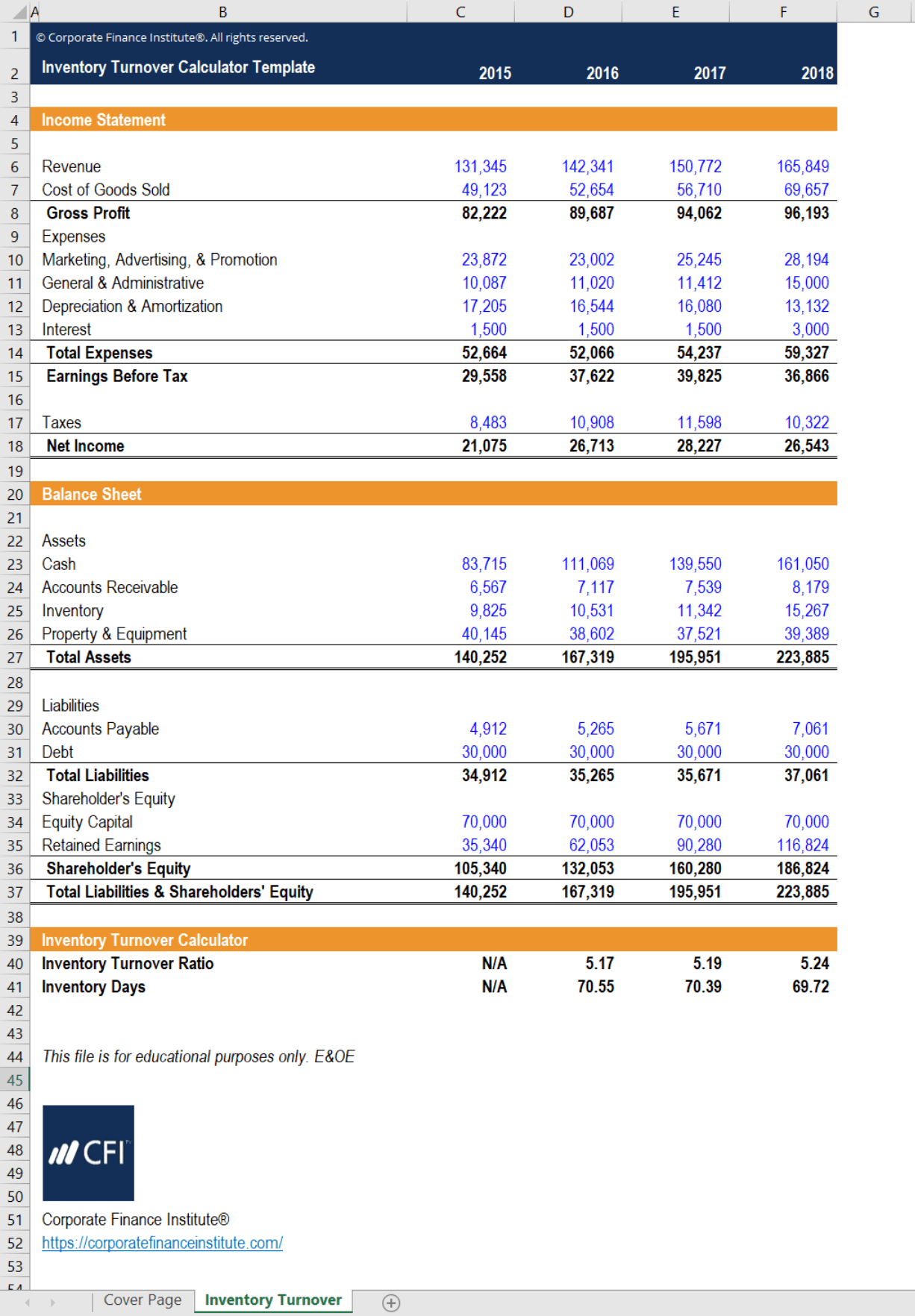# Inventory Turnover Calculator

## Inventory Turnover Calculator

This inventory turnover calculator will help you calculate the number of times a business sells and replaces its stock of products within a set period.

Below is a preview of CFI’s inventory turnover calculator:### Calculating and Using Inventory Turnover

The inventory turnover ratio is calculated using the formula below. CFI’s inventory turnover calculator pulls data from a sample financial statement and uses this formula to calculate the ratio.The inventory turnover ratio is an important measure in financial modeling. The inventory turnover ratio can be manipulated to give inventory turnover days instead. This is done by taking the inverse of the ratio and multiplying it by the number of days in the time period.

For example, take the following information:

• Cost of Goods Sold: \$300,000
• Average Inventory: \$60,000
• # of Days in Period: 365

With this, we calculate 60,000/300,000*365 = 73 days. This means that on average, it takes 73 days for the company to sell an entire stock of inventory. However, an alternative use is that we can make assumptions on inventory turnover days to forecast future inventory.

For example, consider a company who consistently has inventory turnover days of around 62. This company has no plans to expand warehouse operations. Thus, it is relatively safe to assume that inventory held will not change and that inventory turnover days will remain constant into the future. Using the forecast numbers, we can manipulate the formula into Average Inventory = Inventory Turnover Days*Cost of Goods Sold/365. This would allow us to calculate the average inventory and thus the inventory for the forecast year.

Additionally, by knowing how quickly this company sells a stock of inventory, we can assess how well it meets market demand and how its sales compare to other companies. A higher inventory turnover ratio (or lower inventory days) shows efficiency in sales and cost savings from holding lower levels of inventory.

### More Free Templates

For more resources, check out our business templates library to download numerous free Excel modeling, PowerPoint presentation and Word document templates.

• Excel Modeling Templates
• PowerPoint Presentation Templates
• Transaction Document Templates
• CFI Template Marketplace

### Financial Analyst Certification

Become a certified Financial Modeling and Valuation Analyst (FMVA)® by completing CFI’s online financial modeling classes and training program!